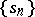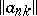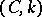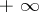# Regular summation methods

(diff) ← Older revision | Latest revision (diff) | Newer revision → (diff)

permanent summation methods

Methods for summing series (sequences) that sum every convergent series (sequence) to the same sum as that to which it converges. Regular summation methods are a special case of conservative summation methods, which sum every convergent series (sequence) to a finite sum, although possibly different from that to which it converges. If a regular summation method is defined by the transformation of a sequenceto a sequenceby means of an infinite matrix:(*)

(see Matrix summation method), then the transformation (*) and the matrix of this transformation,, are called regular.

Many of the most common summation methods are regular. This applies to the Cesàro summation methodsfor, the Hölder summation methods and the Abel summation method, among others. There are non-regular summation methods, such as the Cesàro summation methodfor, and the Riemann summation method.

A summation method is called completely regular if it is regular and if it sums every series (sequence) with real terms converging to(or) to(respectively,). A regular summation method defined by a positive matrix is completely regular (see also Regularity criteria).

How to Cite This Entry:
Regular summation methods. Encyclopedia of Mathematics. URL: http://encyclopediaofmath.org/index.php?title=Regular_summation_methods&oldid=15873
This article was adapted from an original article by I.I. Volkov (originator), which appeared in Encyclopedia of Mathematics - ISBN 1402006098. See original article